Theory

Light Collection

For you to see a star, the light from the star has to get into your eye, and it gets in through the pupil. So the larger the pupil, the more light gets in, and the fainter are stars your eye can detect. While everyone is different, typically the pupil of the eye, when it is adapted to the dark, is about 7 mm in diameter. Click here to see how the dark-adapted pupil varies with age.

What the telescope does is to collect light over a much wider area than just the 7mm of your eye pupil. It then focuses that light down to the size of your eye pupil so you end up with much more light passing into your eye. This enables you to see much fainter stars with a telescope than you could without.Light Grasp

How much more light does the telescope collect? That is known as the "light grasp", and can be found quite simply as the increase in area that you gain in going from using the pupil of your eye to using the objective lens (or mirror) of the telescope.

The area of a circle is found as π/4 × D2, so the light grasp -- we'll call it GL -- is the ratio of the area of the objective to the area of the pupil of the eye, which is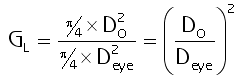Star Brightness and the Magnitude Scale

Astronomers measure star brightness using "magnitudes". The magnitude scale originates from a system invented by the ancient Greeks, where the brightest stars were stars of the first magnitude, like 'first class', and the faintest stars you could see were stars of the sixth magnitude.

When astronomers got telescopes and instruments that could measure star brightness, they found 1st magnitude stars were almost exactly 100 times the brightness of 6th magnitude stars.

You might have noticed this scale is upside-down: the brightest stars get the lowest magnitude numbers, and the faintest stars get the highest numbers. Apparently that sounded like a pretty good idea to the astronomy community, because they decided to fit a logarithmic scale recreating the Greek magnitude system so you can calculate a star's magnitude from its brightness. More accurately, the scale lets you find the magnitude difference between two stars based on the ratio of their brightness using the formula

Magnitude Difference = 2.5 × log(I0/I1).

Where I0 is a reference star, and I1 is the brightness of the star whose magnitude we're calculating. Not so hard, really. Now if I0 is the brightness of a first magnitude star, and I1 is 100 times smaller, then the logarithm will come out to be 2. The formula says multiply that by 2.5, so we get 2.5×2 = 5, which is the difference from the first magnitude star. So to get the magnitude of the fainter star we add that 5 to the "1" of the first magnitude star, resulting in a magnitude 6 which is where we wanted to be. So the scale works as intended.

The scale then sets the star Vega as the reference point, so every star's magnitude is based on it's brightness relative to Vega using the formula above, with I0 set to the brightness of Vega. In fact, if you do the math you would figure out that this means Vega has a magnitude of zero... which is the case, and it says that Vega is brighter than a 1st magnitude star. Not only that, but there are a handful of stars that are brighter than Vega and have negative magnitudes. The brightest star in the sky is Sirius, with a magnitude of -1.5. Well... what is really the brightest star in the sky? The sun has a magnitude of -27.

The Scope's Star Magnitude Limit

Ok so we were supposed to be talking about your telescope so let's get back to that. We've already worked out the brightness increase we get from the scope as GL = (DO/Deye)², so all we need to do is take 2.5×log(GL) and we have the brightness increase of the scope in terms of magnitudes, so it's just Gmag = 2.5×log((DO/Deye)²).

We can take advantage of the logarithm in the equation to simplify it, by making use of the fact that log(x²) = 2×log(x). So then: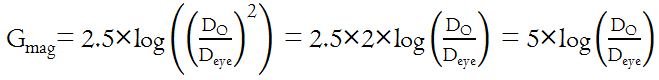When you divide by a number you subtract its logarithm, so subtracting the log of Deye from DO , then substituting 7mm for Deye , we get: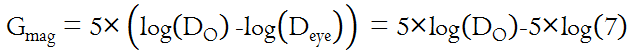Since log(7) is about 0.8, then 5×0.8 = 4 so our equation for the gain in star magnitude isThis represents how many more magnitudes the scope lets me see, over and above what my eye alone can see. Then to find the faintest magnitude I can see in the scope, we simply add Gmag to the faintest magnitude our eye can see, magnitude 6. This is the magnitude limit of the scope, Lmag: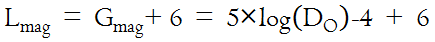Which simplifies down to our final equation for the magnitude limit Lmag of the scope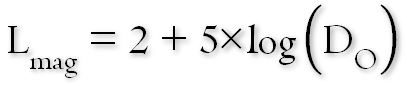By the way did you notice through all this, that the magnitude limit of the scope — the faintest star I can see in the scope — depends only on the diameter of the objective? Interesting result, isn't it?

Immune to MagnificationStars are so ridiculously far away that no matter how massive or blown out of proportion they may be, to us they look like points. Magnify a point, and it's still just a point.

That means that, unlike objects that cover an area, the light from a star does not get spread out as you magnify the image. All the light from the star stays inside the point.

However... as you increase magnification, the background skyglow does get spread out, which means the background gets darker and the star stays bright. This is a nice way of increasing the contrast on stars, and sometimes making fainter stars more visible.

Just remember, this works until you reach the maximum magnification of the scope, which is the same number as the diameter of the scope in millimeters. When you exceed that magnification (or the limits of the atmosphere), the stars start to spread out and dim down just like everything else.

Practice

Which Scope?

I want to go out tonight and find the asteroid Melpomene, which is wandering through Cetus at magnitude 8.6 as I write this. It's just that I don't want to lug my heavy scope out if I can grab my smaller scope (which sits right by the front door at all times) and spot it with that. So the question is -- can I see Melpomene with my 90mm ETX?

I apply the magnitude limit formula for the 90mm ETX, in the hopes that the scope can see better than magnitude 8.6. So the magnitude limit is

Lmag = 2 + 5×log(DO) = 2 + 5×log(90) = 2 + 5×1.95 = 11.75.

Outstanding. I can see it with the small scope. The magnitude limit formula just saved my back.

Setting Software Limits for Star Magnitude

Where I use this formula the most is when I am searching for a deep sky object and want to see how the star field will look in the eyepiece. I can do that by setting my astronomy software to show star magnitudes down to the same magnitude I will be able to see in the telescope.

From my calculation above, I set the magnitude limit for "faintest" stars to 11.75 and the software shows me the star field I will see in the eyepiece. This helps me to identify the asteroid as the "star" that isn't supposed to be there.

Let's suppose I need to see what the field will look like through the viewfinder scope, so I want to find the magnitude limit for the viewfinder. Written right on my viewfinder it says "8x25mm", so the objective of the viewfinder is 25mm, and the magnitude limit is 2 + 5×log(25) = 2 + 5×1.4 = 9. So I would set the star magnitude limit to 9 and the software shows me the star field that I will see through the viewfinder.